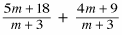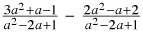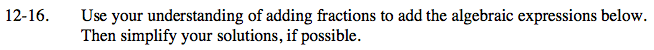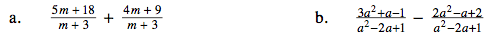### Home > CAAC > Chapter 12 > Lesson 12.1.2 > Problem12-16

12-16.

1.2.Because the denominators are the same, you can add the numerators together.

Factor 9 out of the numerator and simplify the equation by looking for factors that make 1.

9

Subtract the numerators, then try factoring.
Be sure to distribute the negative when performing the subtraction.

$\frac{(3a^2+a-1)-(2a^2-a+2)}{a^2-2a+1}$

Look for factors that make 1.